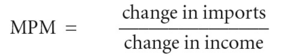# marginal propensity to import

Also found in: Wikipedia.

## marginal propensity to import (MPM)

the fraction of any change in NATIONAL INCOME that is spent on IMPORTS:Alternatively, the change in imports can be expressed as a proportion of the change in DISPOSABLE INCOME. See PROPENSITY TO IMPORT, MULTIPLIER.

Collins Dictionary of Economics, 4th ed. © C. Pass, B. Lowes, L. Davies 2005
References in periodicals archive ?
The signs on the terms in the denominator tell us that an increase in the marginal propensity to consume or invest will increase the multiplier while an increase in the marginal propensity to import will decrease it.
Imports of intermediate goods have raised the marginal propensity to import to 0.49 per dollar rise of GDR This value is significantly high and has to be taken into consideration despite the lack of a foreign exchange gap in the economy (explained by the country's accumulated foreign reserves).
This phenomenon has to be observed closely because it may trigger a process of "high income-led growth" for the consumption of imported, high-value articles that may be beneficial in the short term but will result in serious limitations in the medium term due to the high marginal propensity to import induced by higher income groups.
327.) However, unless there is a total ban on a nation's foreign imports, as y increases the marginal propensity to import will worsen the trade balance.
becomes the higher the marginal propensity to import out of additional GDP.
where a and b are constants, c is the marginal propensity to consume (MPC) and m is the marginal propensity to import (MPM).

Site: Follow: Share:
Open / Close